• 2021-08-14 18:00:29

## 普通直角三角形

思路

• 第一行1个，第二行2个，第三行3个，一此内推，第n行比第n-1行多一个，要有两个循环语句，第一个循环代表行数，第二次循环画出三角形的轮廓，第一行画一个，第二行画两个，总之画出的图形要小于等于行数。

代码

public class dome2{
public static void main(String[] args) {
for(int i=1;i<=5;i++) {    //代表行
for(int j=1;j<=i;j++) {   //代表列，画出图形
System.out.print("*");
}
System.out.println();  //换行
}
}
}


答案
*
**
***
****
*****

## 等腰直角三角形

思路

• 跟直角三角形的排列方式差不多，总比前一行多一个，等腰三角形的左边有一个空倒立的三角形，专门为等腰三角形导出形状，需要三个循环语句，一个循环最左边空形状倒立的三角形，把等腰三角形分成两个直角三角形，第二个循环左边的直角三角形，第三个循环右边的直角三角形。

代码

public class dome2{
public static void main(String[] args) {
for(int i=1;i<=7;i++) {    //一共7行
for(int j=6;j>=i;j--) {
System.out.print(" "); //左边的空倒立三角形
}
for(int j=2;j<=i;j++) {
System.out.print("*");  //左边被分割的直角三角形
}
for(int j=1;j<=i;j++) {
System.out.print("*"); //右边的被分割直角三角形
}
System.out.println();
}
}
}


答案

      *
***
*****
*******
*********
***********
*************



# 杨辉三角形

思路

• 每行前面都有空格，并且每一行空格都在缩小，这需要根据行数来判断，每一行中间的数值是与前一行对齐相邻中间数的和，可以根据前面的值来算出。

代码

public class dome2{
public static void main(String[] args) {
play(5);

}
public static void play(int row) {
for (int i = 1; i <= row; i++) {    //输出行数
for (int j =1; j<=row-i; j++) {    //输出左边空格的形状
System.out.print(" ");
}
for (int j = 1; j <= i; j++) {    //调用nve函数输出三角形
System.out.print(nve(i, j) + " ");
}
System.out.println();    //换行
}
}
public static int nve(int x, int y) {
if (y == 1 || y == x) {
return 1;
}
int c = nve(x - 1, y - 1) + nve(x - 1, y);
return c;
}
}

    1
1 1
1 2 1
1 3 3 1
1 4 6 4 1


# 空三角形

思路

• 与上面的杨辉三角形差不多，只是边上才有图，中间的算法直接省去，改成空字符串。

代码

public class dome2{
public static void main(String[] args) {
play(6);

}
public static void play(int row) {
for (int i = 1; i <= row; i++) {    //输出行数
for (int j =1; j<=row-i; j++) {    //输出左边空格的形状
System.out.print(" ");
}
for (int j = 1; j <= i; j++) {    //调用nve函数输出三角形
System.out.print(nve(i,j,row) + " ");
}
System.out.println();    //换行
}
}
public static String nve(int x,int y,int tt) {
if((y==1||y==x)&&x!=tt) {  //在第一列和最后一列输出，最后一样必须全部输出
return "*";
}else if(x==tt) {  //最后一行全部输出
return "*";
}else {
return " ";
}
}
}

     *
* *
*   *
*     *
*       *
* * * * * *

更多相关内容
• ## java输出数字三角形

千次阅读 2017-06-16 00:02:22
public class MyTest { /** * 输出数字三角形 * @param n 三角形的高 */ private static void printTriangle(int n){ for(int i=0;i;i++){ for(int y=i;y;y++){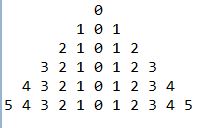public class MyTest {
/**
* 输出数字三角形
* @param n 三角形的高
*/
private static void printTriangle(int n){
for(int i=0;i<=n;i++){
for(int y=i;y<n;y++){
System.out.print("  ");//输出两个空格
}
for(int y=i;y>=-i;y--){
System.out.print(Math.abs(y)+" ");//输出数字
}
System.out.println();
}
}
public static void main(String[] args) {
MyTest.printTriangle(5);
}
}

展开全文• ## java输出三角形

千次阅读 2022-02-07 15:58:32
} } } 输出结果为： public class Demo3 { /**利用循环打印输出一个三角形▲ * 三角形行数自定义**/ public static void main(String[] args) { //外循环控制行数：5行 for (int i=1;i;i++) { for (int j=5;j>=i;j-...
public class Demo1 {
public static void main(String[] args)
{
//外循环控制行数：5行
for (int i=1;i<=5;i++) {
for (int j = 1; j <= i; j++) {
System.out.print("*");
}
//换行
System.out.println();
}
}
}


输出结果如下：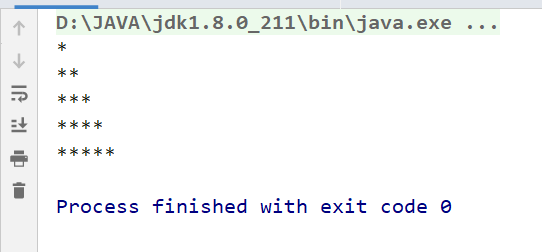public class Demo2 {
public static void main(String[] args)
{
//外循环控制行数：5行
for (int i=1;i<=5;i++) {
//循环控制输出空格
for (int j = 5; j >= i; j--) {
System.out.print(" ");
}

for (int a=1;a<=i;a++)
{
System.out.print("*");
}
//换行
System.out.println();
}
}
}


输出结果为：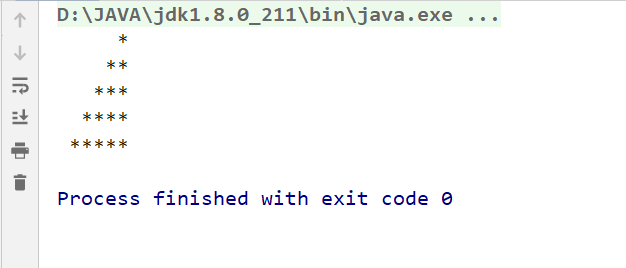public class Demo3 {
/**利用循环打印输出一个三角形▲
* 三角形行数自定义**/
public static void main(String[] args)
{
//外循环控制行数：5行
for (int i=1;i<=5;i++)
{
for (int j=5;j>=i;j--)
{
System.out.print(" ");
}
for (int a=1;a<=i;a++)
{
System.out.print("*");
}
for (int c=1;c<i;c++)
{
System.out.print("*");
}
System.out.println();
}
}
}


运行结果：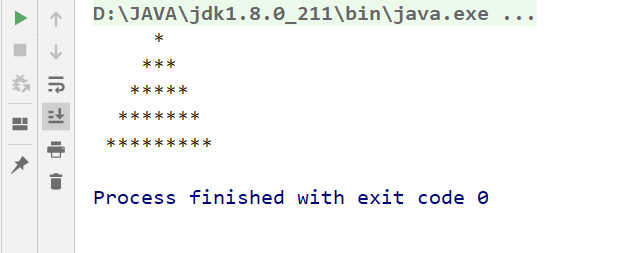展开全文开发语言 后端
• 输入指定行数：输出类似如下图形 想过用循环但做不出来。 最后的效果图如下： 1*** 2*** 3*** 4*** 5*** 6*** 7*** 8*** 9*** 10** 11** 12** 13** 14** 15***开发语言
• 输入一个数字 n（2~9）输出数字三角形 import java.util.Scanner; public class SanJiao { public static void main(String[] args) { Scanner input = new Scanner(System.in); System.out.println("输出数字...

输入一个数字 n（2~9）输出数字三角形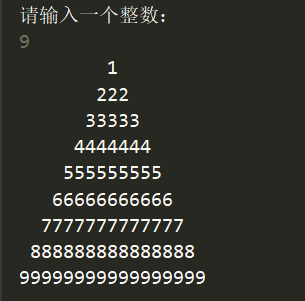import java.util.Scanner;

public class SanJiao {
public static void main(String[] args) {
Scanner input = new Scanner(System.in);
System.out.println("输出数字三角形");
System.out.println("请输入一个整数：");
int a = input.nextInt();
for (int i = 1; i <= a; i++) {
for (int j = 1; j <= a-i; j++) {
System.out.print(" ");
}
for (int k = 1; k <= 2*i-1; k++) {
System.out.print(i);
}
System.out.println();
}
}
}

展开全文算法 编程语言
• >按照对角线的方式将数字放入数组中,从1到n.代码中的s表示x y sum.每个对角线的总和是恒定的.在第一对角线(最长的一个)中,和等于n-1.在第二对角线中,和为1,n.在最后的“对角线”(右下角)中,总和为2 *(n – 1).这...
• 输入一个整数n(2~9),输出含n行的数字三角形。如下是n=4的三角形。 1 222 33333 4444444 import java.util.Scanner; public class NumTriangle{ public static void main(String[] arg){ System.out.print(...
• public class 直角三角形 { /** * @param args */ public static void main(String[] args) { // TODO Auto-generated method stub for(int a=1; a<=9;a++){ for(int b=1; b<=a;b++){ System.out.pr....
• Java输出杨辉三角形 一、杨辉三角的概念 杨辉三角是二项式系数在三角形中的一种几何排列，南宋数学家杨辉在《详解九章算法》中有记载，欧洲的帕斯卡也发现这一规律，故又称帕斯卡三角形，比杨辉晚几百年发现该规律...
• public class Demo { /* 注意：编程时不可以使用汉语和拼音，要用英文单词或者英文单词缩写 如图,想要输出一个四行的三角形： 第1行 * 第2行 *** 第3行 ***** 第4行 ******* 思路.
• } } } 二：（靠左侧） 与输出矩形不同的一点时，如果要输出等腰直角三角形的话，要控制每一行每一列的*数与该行数字相同。 因此，j正常循环，表示输出三角形的行数。 那么要保证i要与j一致，所以在嵌套的循环中，...数据结构
• 给定一个如下图所示的数字三角形，从顶部出发，在每一结点可以选择移动至其左下方的结点或移动至其右下方的结点，一直走到底层，要求找出一条路径，使路径上的数字的和最大。 7 3 8 8 1 0 2 7 4 4 4 5 2 6 5 ...
• 题目：输入一个数字n，输出一个n层的特定三角形三角形数字增长是有规律的 代码如下，基本能满足要求。抛砖引玉，希望能给小伙伴们点启发 #!/usr/bin/env python3 #-*- coding:utf-8 -*- num = int(input('请...
• 在键盘上输入两个数m和n，m为行数，n为第一个数，按以下两种情况输出例：当m=4，n=3时(n=3为奇数)当m=3，n=4时(n=4为偶数)3445656789879123最好有详细一点的解释谢谢现在我的程序...在键盘上输入两个数m和n，m为行数...
• ## Java输出直角三角形方法

万次阅读 多人点赞 2018-10-30 20:42:39
//打印一个正直角三角形。 public class testfor{ public static void main(String[] args){  for(int i=1;i;i++){  for(int j=1;j;j++){  System.out.print("*");  }  System.out.println();  }  } } ...
• 蓝桥杯历届真题解析Java版- 数字三角形 - 2020省赛算法 动态规划
•算法
• 展开全部纯粹的for循环，望采纳！intcount=0;Scannersc=newScanner(System.in);System.out.println("输入层数e69da5e887aa3231313335323631343130323136353331333330353538：");count=sc.nextInt();...
• Java输出等腰三角形 import java.util.Scanner; /** * 输出等腰三角形 */ public class hello { public static void main(String[] args){ Scanner sc = new Scanner(System.in); System.out.print("输入...
• 打印正直角三角形，代码如下： public class Dome2 { public static void main(String[] args) { //星星行数 for (int i = 0; i < 5; i++) { //星星个数 for (int j = 0; j <= i; j++) { ...开发语言 后端
• 1、实现直角三角形效果： 源代码：package square;public class SanJiao {public static void main(String[] args) {int i, j;for (i = 0; i < 10; i++) {for (j = 0; j < 9 - i; j++) {// 打印空格System.out...
• 接下来 n 行，每行包含若干整数，其中第i行表示数字三角形第i层包含的整数。 输出格式 输出一个整数，表示最大的路径数字和。 数据范围 1≤n≤500 //数据范围要注意，开的数组下标必需够用，否则部分样例是无法...
• import java.sql.SQLOutput;...//输出一个正三角形 public class Test1 { public static void main(String[] args) { Scanner input = new Scanner(System.in); System.out.print("请输入行数"); int.开发语言
• 问题描述 5 7 3 8 8 1 0 2 7 4 4 4 5 2 6 5 ...上图给出了一个数字三角形。从三角形的顶部到底部有很多条不同的路径。对于每条路径，把路径上面的数加起来可以得到一个和，你的任务...数字三角形上的数都是 0 至 10.算法 动态规划 后端
• Java-数字三角形(递归法) 题目描述： 上图给出了一个数字三角形。从三角形的顶部到底部有很多条不同的路径。对于每条路径，把路径上面的数加起来可以得到一个和，你的任务就是找到最大的和。 路径上的每一步只能从...算法 数据结构
• public class Demo { public static void main (String... System.out.println("输入三角形行数:"); Scanner s= new Scanner(System.in); int n= s.nextInt(); for (int a = 0 ; a <=n ;a++){ f...
• 　输出一个n行的与样例类似的数字三角形,必须使用递归来实现 输入格式 　一个正整数数n，表示三角形的行数 输出格式 　输出一个与样例类似的n行的数字三角形，同一行每两个数之间用一个空格隔开即可(图中只是为...递归
• 　（图３.１－１）示出了一个数字三角形。 请编一个程序计算从顶至底的某处的一条路 　径，使该路径所经过的数字的总和最大。 　●每一步可沿左斜线向下或右斜线向下走； 　●1三角形行数≤100； 　●三角形中的数字...算法 dfs...

# java输出数字三角形java 订阅